# Tangent Lines Understanding Tangent Lines A tangent to

• Slides: 22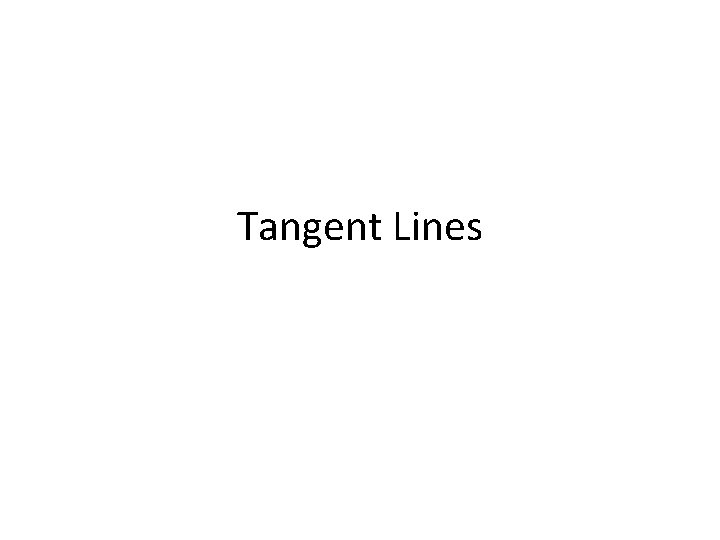Tangent Lines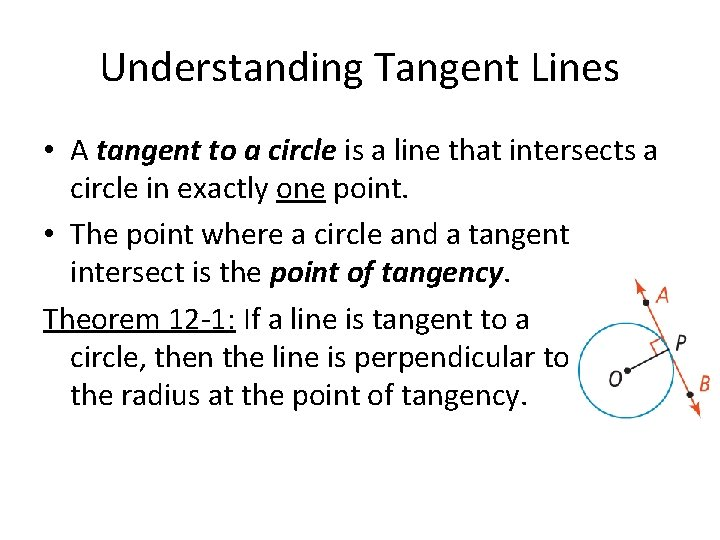Understanding Tangent Lines • A tangent to a circle is a line that intersects a circle in exactly one point. • The point where a circle and a tangent intersect is the point of tangency. Theorem 12 -1: If a line is tangent to a circle, then the line is perpendicular to the radius at the point of tangency.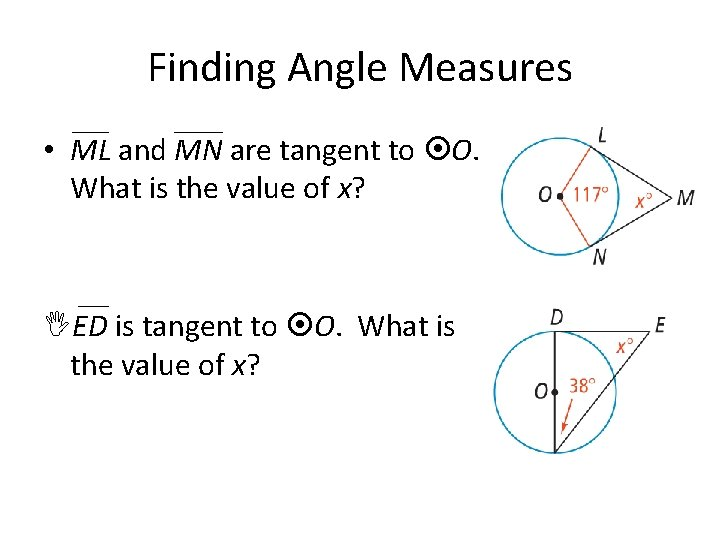Finding Angle Measures • ML and MN are tangent to O. What is the value of x? ED is tangent to O. What is the value of x?Finding a Radius • What is the radius of C? What is the radius of O?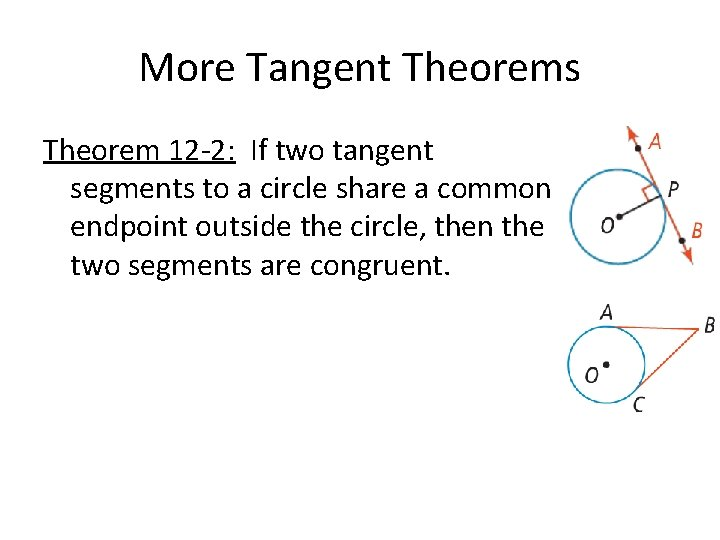More Tangent Theorems Theorem 12 -2: If two tangent segments to a circle share a common endpoint outside the circle, then the two segments are congruent.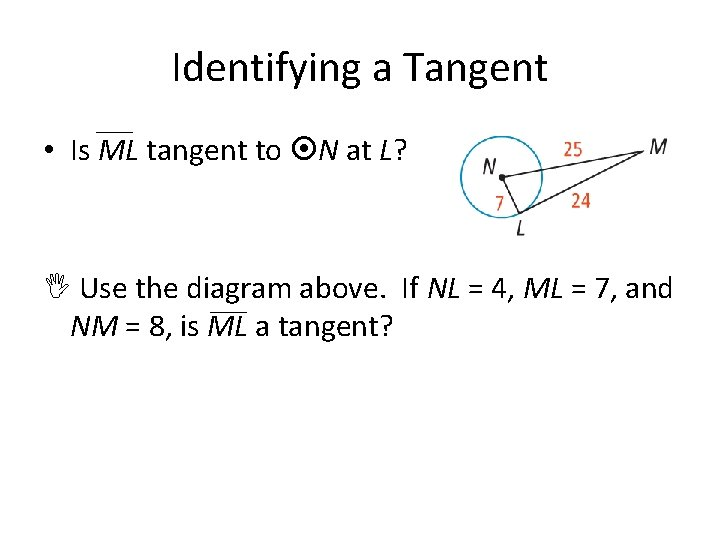Identifying a Tangent • Is ML tangent to N at L? Use the diagram above. If NL = 4, ML = 7, and NM = 8, is ML a tangent?Circles Inscribed in Polygons • What is the perimeter of ΔABC? If the perimeter of ΔPQR is 88 cm, what is QY?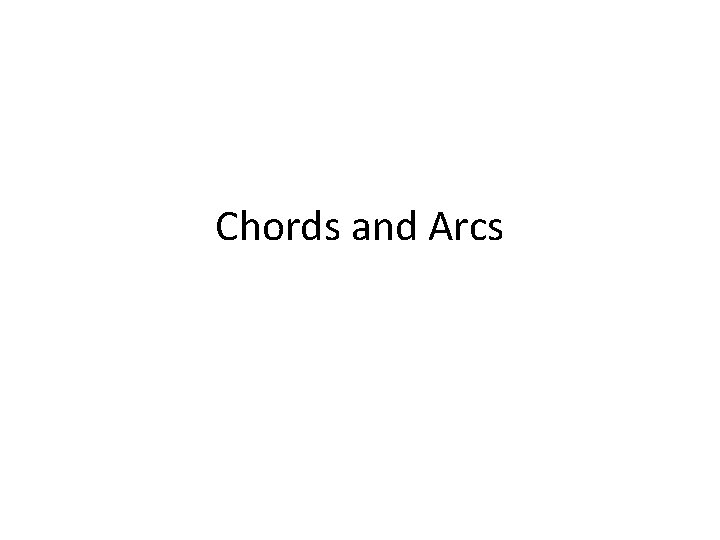Chords and Arcs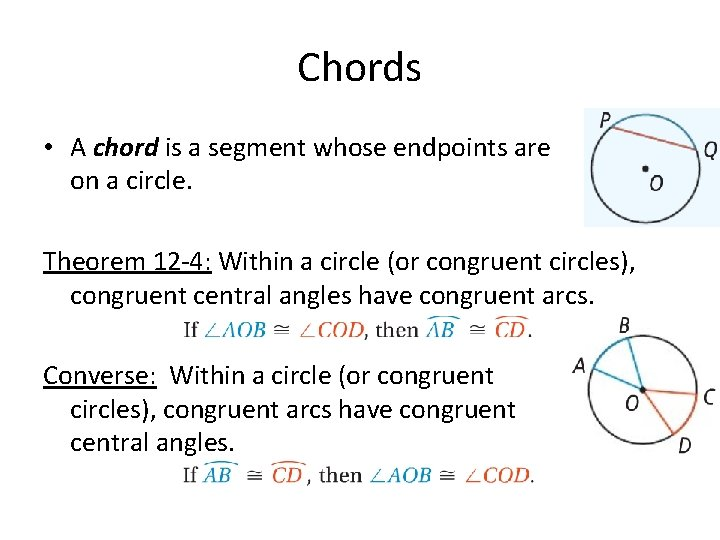Chords • A chord is a segment whose endpoints are on a circle. Theorem 12 -4: Within a circle (or congruent circles), congruent central angles have congruent arcs. Converse: Within a circle (or congruent circles), congruent arcs have congruent central angles.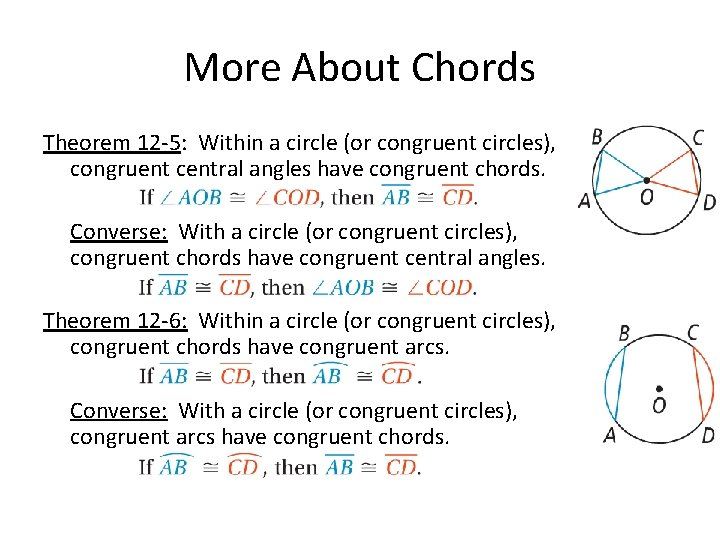More About Chords Theorem 12 -5: Within a circle (or congruent circles), congruent central angles have congruent chords. Converse: With a circle (or congruent circles), congruent chords have congruent central angles. Theorem 12 -6: Within a circle (or congruent circles), congruent chords have congruent arcs. Converse: With a circle (or congruent circles), congruent arcs have congruent chords.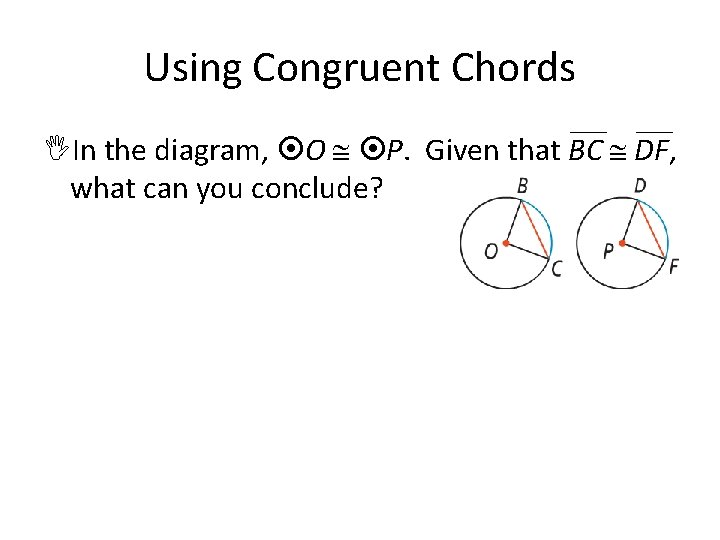Using Congruent Chords In the diagram, O P. Given that BC DF, what can you conclude?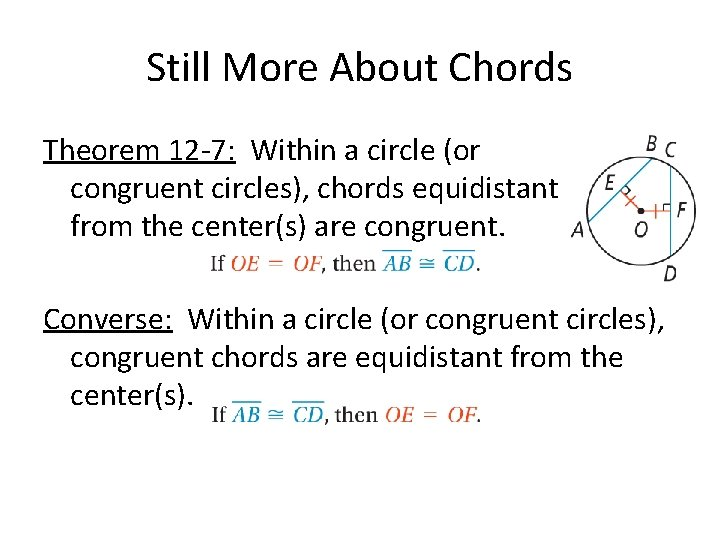Still More About Chords Theorem 12 -7: Within a circle (or congruent circles), chords equidistant from the center(s) are congruent. Converse: Within a circle (or congruent circles), congruent chords are equidistant from the center(s).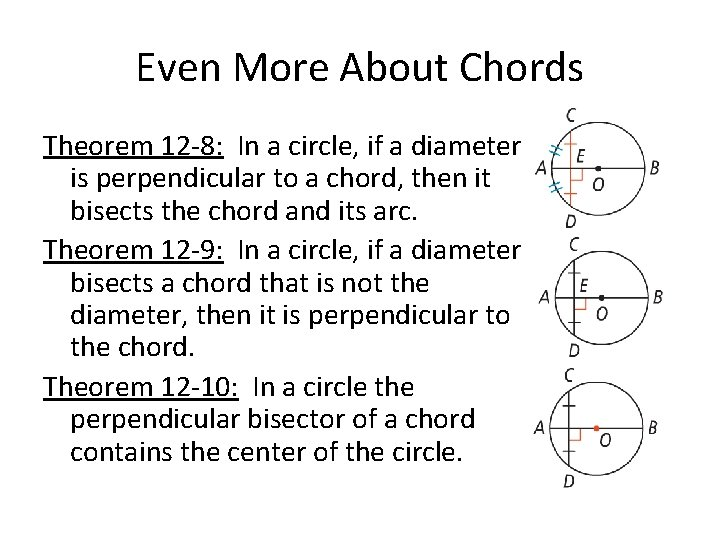Even More About Chords Theorem 12 -8: In a circle, if a diameter is perpendicular to a chord, then it bisects the chord and its arc. Theorem 12 -9: In a circle, if a diameter bisects a chord that is not the diameter, then it is perpendicular to the chord. Theorem 12 -10: In a circle the perpendicular bisector of a chord contains the center of the circle.Finding the Length of a Chord • What is RS in O? What is the value of x?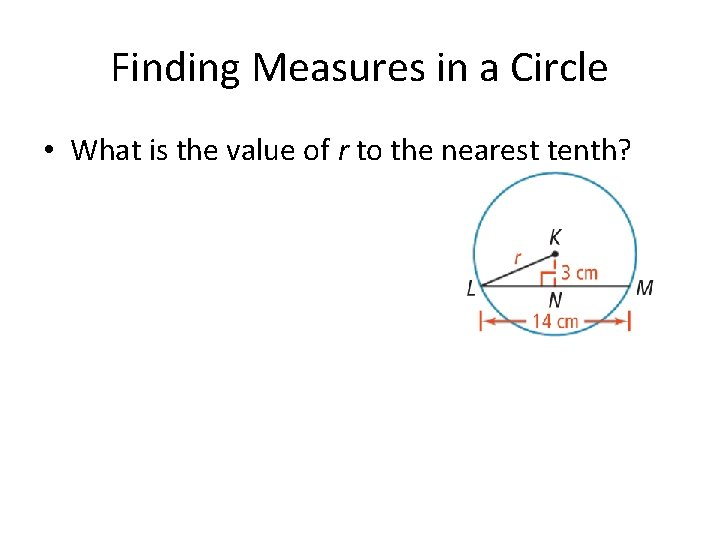Finding Measures in a Circle • What is the value of r to the nearest tenth?Solve for x, QT UV = 6; RS = 3; ST = 3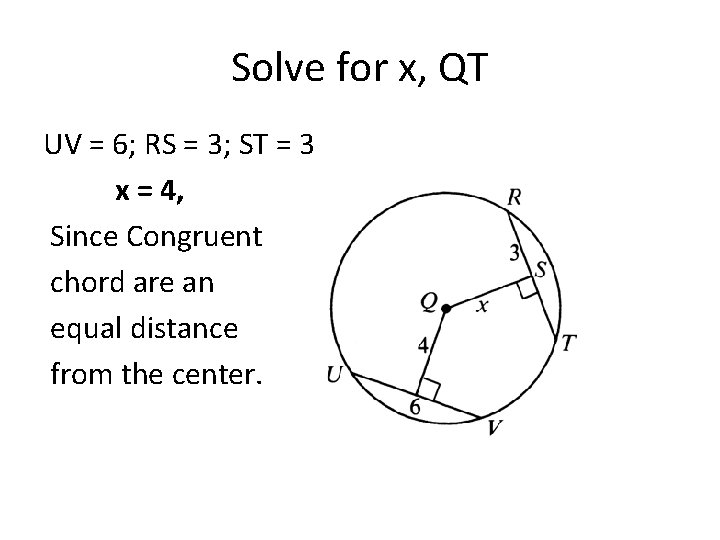Solve for x, QT UV = 6; RS = 3; ST = 3 x = 4, Since Congruent chord are an equal distance from the center.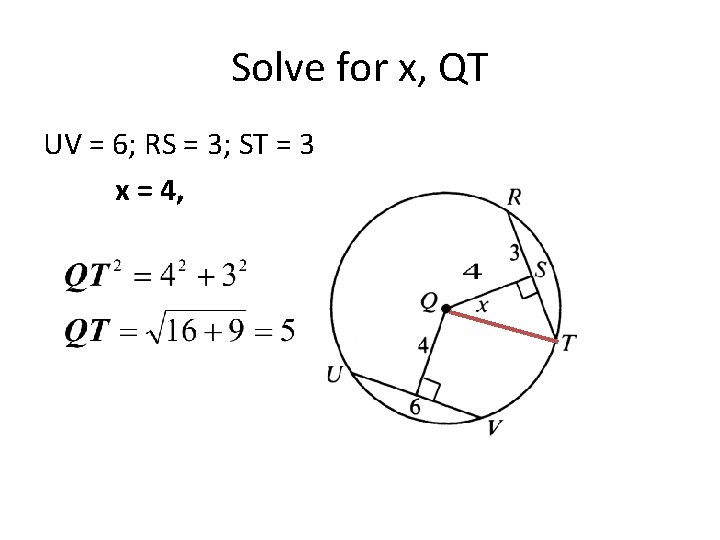Solve for x, QT UV = 6; RS = 3; ST = 3 x = 4,Find the measure of the arc Solve for x and y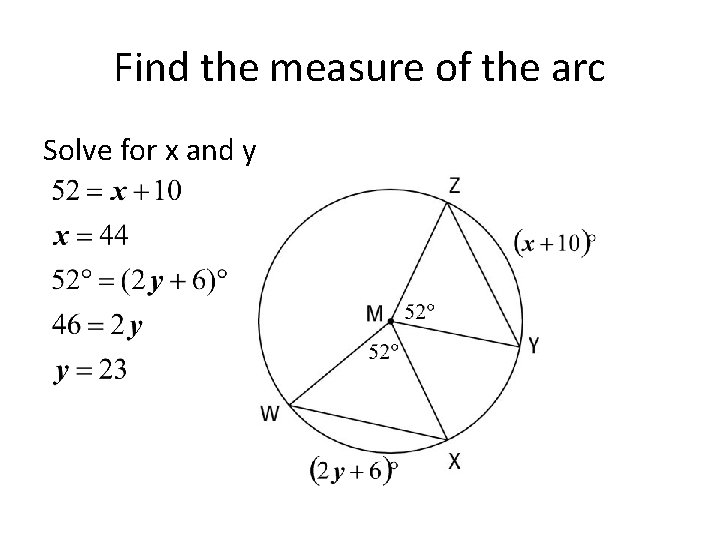Find the measure of the arc Solve for x and y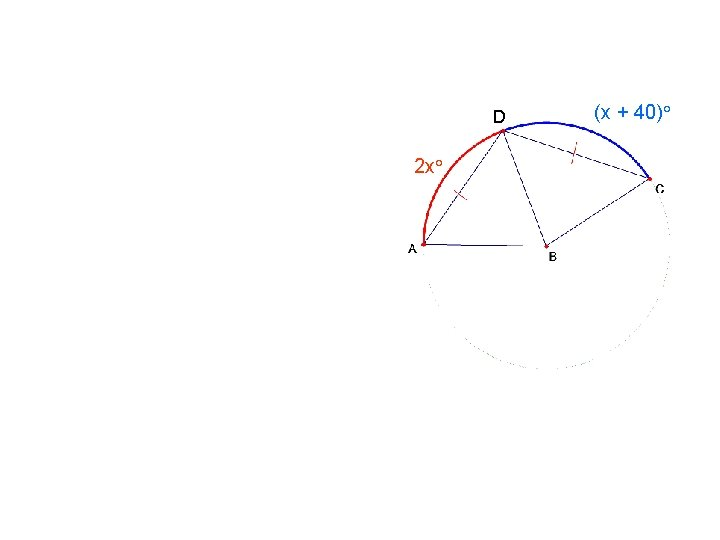D 2 x° (x + 40)°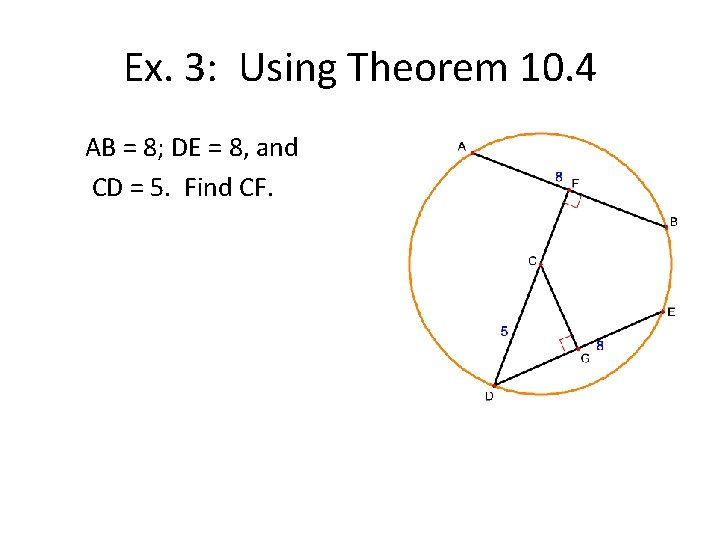Ex. 3: Using Theorem 10. 4 AB = 8; DE = 8, and CD = 5. Find CF.